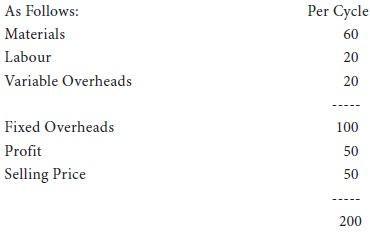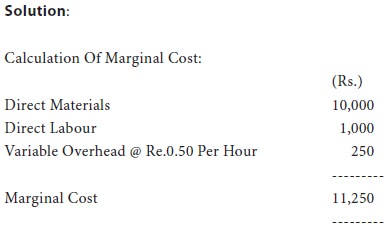Home | ARTS | Accounting For Managers | Pricing Decisions-Application Of Marginal Costing

# Pricing Decisions-Application Of Marginal Costing

Posted On :  03.05.2018 07:10 am

Marginal costing techniques help a firm to decide about the prices of various products in a fairly easy manner. Let’s examine the following cases:

Pricing Decisions

Marginal costing techniques help a firm to decide about the prices of various products in a fairly easy manner. Let’s examine the following cases:

## (I)  Fixation of Selling Price

### Illustration 12:

P/V Ratio Is 60% and the marginal cost of the product is Rs.50. What will be the selling price?## (ii)  Reducing Selling Price

### Illustration 13:

The Price Structure Of A Cycle Made By The Visu Cycle Co. Ltd. IsThis is based on the manufacture of one lakh cycles per annum. The company expects that due to competition they will have to reduce selling prices, but they want to keep the total profits intact. What level of production will have to be reached, i.e., how many cycles will have to be made to get the same amount of profits, if:
(a)   the selling price is reduced by 10%?

(b)   the selling price is reduced by 20%?

 Solution: (Rs.) (Rs.) Existing profit = 1,00,000 x 50 = 50,00,000 Total fixed overheads = 1,00,000 x 50 = 50,00,000

(a)    Selling price is reduced by 10% and to get the existing profit of rs.50 lakhs.
 New Selling Price = 200 – 10% Of Rs.200 = 200 – 20 =Rs.180 New Contribution = 180 – 100 =Rs.80 Per Unit Total Sales (Units) = F + P/Contribution Per Unit 5,00,000 + 5,00,000 = --------------------------- 80 = 1,25,000 Cycles

Are to be obtained and sold to earn the existing profit of rs.5,00,000.

(b) Selling price reduced by 20% and to get the existing profit of rs.5,00,000.

 New Selling Price = 200 – 20% Of Rs.200 = 200 – 40 = Rs.160 New Contribution = S – V = 160 – 100 = Rs.80 Per Unit Total Sales (Units) = F + P/Contribution Per Unit 5,00,000 + 5,00,000

=               ------------------------------------------

60

=                    1,66,667 cycles are to be produced

and sold to earn the existing profit of rs.50 Lakhs.

## (iii)  Pricing During Recession:

### Illustration 14:

SSA company is working well below normal capacity due to recession. The directors of the company have been approached with an enquiry for special job. The costing department estimated the following in respect of the job.

Direct Materials                                                                  Rs.10,000

Direct Labour 500 Hours @                                                 Rs.2 Per Hour

Variable                                                                              Re.0.50 Per Hour

Fixed                                                                                  Re.1.00 Per Hour

The directors ask you to advise them on the minimum price to be charged.

Assume that there are no production difficulties regarding the job.Commentary:

Here the minimum price to be quoted is Rs.11,250 which is the marginal cost. By quoting so, the company is sacrificing the recovery of the profit and the fixed-costs. The fixed costs will continue to be incurred even if the company does not accept the offer. So any price above Rs.11,250 is welcome.

Tags : Accounting For Managers - Management Accounting-Marginal Costing
Last 30 days 545 views